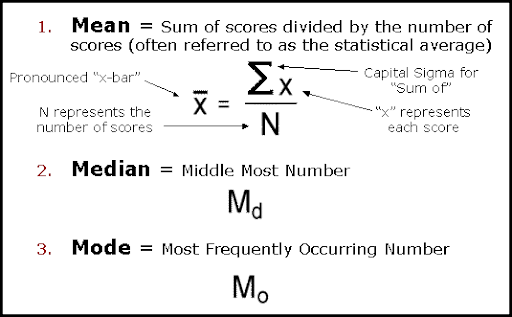# ICAR ARS NET Model Questions on Agricultural statistics

1. Unit less measure of dispersion is
a) Range
b) Standard deviation
c) Mean deviation
d) CV

2. Which measure of central tendency requires data arrangement in ascending or descending order for its estimation?
a)Arithmetic mean
b)Median
c)Mode
d)Harmonic mean

3. The most frequent occurred value of data or whose frequency is maximum is known as
a)Arithmetic mean
b)Median
c)Mode
d)Harmonic meanPoints to remember: Measure of Central Tendency
4. In a symmetrical distribution of data which pair is correct?
a) Mean = Median=Mode
b) Mean > Median >Mode
c) Mean < Median<Mode
d) Mean = Median>Mode

5. To calculate the average speed, which item of central tendency is most suited?
a)Median
b) Mode
c) Weighted HM
d) Weighed AM

6. Mean deviation is the least when calculated about (minimal property)
a)median
b)mode
c)arithmetic mean
d)geometric mean

7. The hypothesis of committing type I error is known as
a) test of significance
b) level of significance
c) composite hypothesis
d) none of the above

8. For comparison of two means from independent samples which test is applicable
a) F test
b) t test
c) z test
d) chi square test

9. The coefficient of variation can be calculated by using the formula
a) Standard deviation / geometric mean x 100
b) Arithmetic mean /Standard deviation x 100
c) Square root of Standard deviation/ arithmetic mean x 100
d) Standard deviation / arithmetic mean x 100

10. Arithmetic mean and variance are always equal in
a) Binomial
b) Poisson distribution
c) Normal distribution
d) None of the above

1. d) CV
2. b)Median
3. c)Mode
4. a) Mean = Median=Mode
5. c) Weighted HM
6. a)median
7. b) level of significance
8. b) t test
9. d) Standard deviation / arithmetic mean x 100
10. b) Poisson distribution